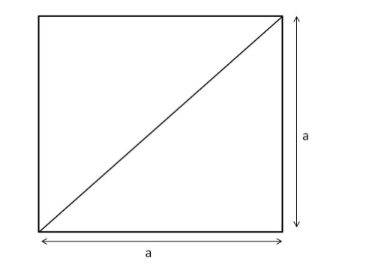# The length of a square field is 0.5 hectare.`
Question:

The length of a square field is 0.5 hectare. The length of its diagonal is

(a) $150 \mathrm{~m}$

(b) $100 \sqrt{2}$

(c) 100 m

(d) $50 \sqrt{2} \mathrm{~m}$

Solution:

(c) 100 m

Disclaimer :- The length cannot be in hectare So we used is as area of the square.

Area of the square field $=0.5 \times 10000=5000 \mathrm{~m}^{2}$The diagonal divides the square into two isosceles right-angled triangles.

Using Pythagoras' theorem, we have:

Diagonal $^{2}=a^{2}+a^{2}=2 a^{2}$

Area of a square $=a^{2}$

$\therefore$ Diagonal $=\sqrt{2 \text { area }}=\sqrt{2 \times 5000}=\sqrt{10000}=100 \mathrm{~m}$